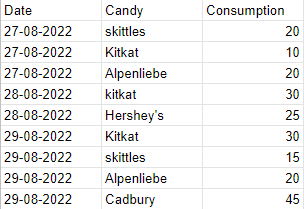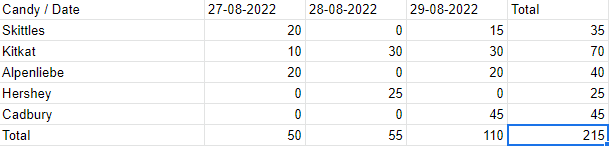Open in App
Not now

# Data Aggregation in Java using Collections Framework

• Difficulty Level : Hard
• Last Updated : 15 Sep, 2022

Let’s look at the problem before understanding what data aggregation is. You are given daily consumption of different types of candies by a candy-lover. The input is given as follows.

Input Table:Data Before Aggregation

Desired Output Table:Data After Aggregation

Above is the aggregated data. Now let’s understand What is Data Aggregation?

What is Data Aggregation?

Let’s understand it with an example. For instance, in the input table, we are given the consumption amount of different kinds of candies each day. For example, on Aug 28, 2022, the volunteer consumed only 2 kinds of candies which are KitKat and Hershey’s. Whereas, on Aug 29, 2022, the volunteer consumed 4 kinds of candies which are KitKat, Skittles, Alpen Liebe and Cadbury. Now, we are also given the consumption amount. For instance, the most eaten candy on Aug 29, 2022, is Cadbury. Whereas, the most eaten candy on Aug 28, 2022, is KitKat. However, looking at the input table, we cannot directly answer the following question: Which candy is the most popular each day (or even which candy is popular overall)?

Now, it seems like looking at the above input table we can answer the question by immediately looking over the data for matching dates. But imagine, we run the survey for a month or a quarter and we now introduce 100 more brands of candies for the volunteer to choose and eat from. The size of the data will grow so quickly, that it would be almost impossible to answer the question just by looking at the table. There’s even another possibility where the data is scattered such that the data collected for a specific date is not shown consecutively as shown in the input table above. In that case, it would become even more complicated to directly look at the raw data and answer.

Now to answer such statistical questions in an efficient manner, we would need to organize our data. We would need to categorize the data in such a way that by looking at our transformed data, we can immediately answer the question that is:- which candy is more popular each day? For instance, by looking at the data after aggregation, we can say that on August 28, KitKat is more eaten and on Aug 29, Cadbury is more eaten just by looking at the column under each date. Not only that, but we can now also answer the following questions:

1. On what date was a particular kind of candy eaten more? (By looking at the row of that candy)
2. Which candy is popular overall? (By looking at the last “Total” column).
3. Which day witnessed the most candy consumption? (By looking at the last “Total” row)

For example,

• Alpenliebe was eaten more on Aug 27 and Aug 29.
• Kitkat on the other hand is the overall popular candy.
• Aug 29, turned out to be the day when most candies were consumed. Maybe, we can declare it “Candy Day”.

So, we are experiencing the benefits of aggregating the data. It’s a technique of summarizing the data we have for the purpose of analyzing it, making the raw data more meaningful. We are now in a more efficient position to answer the above questions.

### Problem Statement

We are required to transform the given input table of candy consumption on a specific date into an aggregated table where data collected for each candy should be aggregated into a value for a day. (Refer to the output table above). Following is the code for the above problem:

## Java

 `import` `java.util.*;`` ` `class` `CandyConsumption {``    ``String date;``    ``String candy;``    ``int` `consumption;`` ` `    ``CandyConsumption(String date, String candy, ``int` `consumption){``        ``this``.date = date;``        ``this``.candy = candy;``        ``this``.consumption = consumption;``    ``}`` ` `    ``public` `String toString(){``        ``StringBuffer str = ``new` `StringBuffer();``        ``str.append( date );``        ``str.append( ``"\t\t\t\t"` `);``        ``str.append( String.valueOf( candy ) );``        ``str.append( ``"\t\t\t\t"` `);``        ``str.append( String.format(``"%20s"``, String.valueOf( consumption ) ));``        ``return` `str.toString() ;``    ``}`` ` `    ``public` `static` `void` `main(String[] args){``        ``CandyConsumption[] cc = ``new` `CandyConsumption[``9``];``        ``cc[``0``] = ``new` `CandyConsumption(``"27-08-2022"``, ``"skittles"``, ``20``);``        ``cc[``1``] = ``new` `CandyConsumption(``"27-08-2022"``, ``"Kitkat"``, ``10``);``        ``cc[``2``] = ``new` `CandyConsumption(``"27-08-2022"``, ``"Alpenliebe"``, ``20``);``        ``cc[``3``] = ``new` `CandyConsumption(``"28-08-2022"``, ``"Kitkat"``, ``30``);``        ``cc[``4``] = ``new` `CandyConsumption(``"28-08-2022"``, ``"Hershey's"``, ``25``);``        ``cc[``5``] = ``new` `CandyConsumption(``"29-08-2022"``, ``"Kitkat"``, ``30``);``        ``cc[``6``] = ``new` `CandyConsumption(``"29-08-2022"``, ``"skittles"``, ``15``);``        ``cc[``7``] = ``new` `CandyConsumption(``"29-08-2022"``, ``"Alpenliebe"``, ``20``);``        ``cc[``8``] = ``new` `CandyConsumption(``"29-08-2022"``, ``"Cadbury"``, ``45``);`` ` `        ``// Before Aggregation``        ``System.out.println(``"Date\t\t\t\t\tCandy\t\t\t\tConsumption"``);``        ``for``( ``int` `i = ``0` `; i < cc.length ; i++ ) {``            ``System.out.println(cc[i]) ;``        ``}`` ` `        ``System.out.println();``        ``System.out.println();``        ``System.out.println(``"After Aggregation"``);``        ``System.out.println();`` ` `        ``// After aggregation``        ``aggregate(cc);``    ``}`` ` `    ``public` `static` `void` `aggregate(CandyConsumption[] cc){``        ``// Key => Candy Column (a/c to output table) | ``        ``// Value = Another HashMap which maps each date ``        ``// to the amount of candies consumed on that date``        ``HashMap> map = ``new` `HashMap<>();`` ` `        ``// An arraylist to store unique dates``        ``ArrayList dates = ``new` `ArrayList<>();`` ` `        ``// HashMap to calculate total consumption datewise``        ``// Key => Date | Value => Total number of ``        ``// candies consumed on that Date``        ``HashMap consumptionDatewise = ``new` `HashMap<>();`` ` `        ``// HashMap to calculate total consumption candywise``        ``// Key => Candy | Value => Total number of candies ``        ``// consumed of that Candy type``        ``HashMap consumptionCandywise = ``new` `HashMap<>();`` ` `        ``// Populate map HashMap``        ``for``(``int` `i=``0``;i());``            ``}`` ` `            ``map.get(candy).put(date, consumption);`` ` `            ``// Let's also populate the dates``            ``// arraylist simultaneously``            ``if``(!dates.contains(date)){``                ``dates.add(date);``            ``}`` ` `            ``// Let's also populate the ``            ``// consumptionDatewise hashmap``            ``if``(!consumptionDatewise.containsKey(date)){``                ``consumptionDatewise.put(date, ``0``);``            ``}`` ` `            ``consumptionDatewise.put(date, consumptionDatewise.getOrDefault(date, ``0``) + consumption);``        ``}`` ` `        ``// We have calculated total consumption datewise. ``        ``// Let's now calculate the total consumption``        ``// of each candy``        ``for``(String candy : map.keySet()){``            ``HashMap candyVal = map.get(candy);``            ``int` `total = ``0``;``            ``for``(String date : candyVal.keySet()){``                ``total += candyVal.get(date);``            ``}``            ``consumptionCandywise.put(candy, total);``        ``}`` ` `        ``// We are done with all the necessary pre-processing. ``        ``// Let's start printing. ``        ``// Let's print the Header Line first ``        ``System.out.print(String.format(``"%-15s"``, ``"Candy/Date"``));``        ``for``(String date : dates){``            ``System.out.print(date + ``"\t"``);``        ``}``        ``System.out.println(``"Total"``);`` ` `        ``// Printing the rest of the table``        ``for``(String candy : map.keySet()){``            ``// System.out.printf("%-4s", candy);``            ``System.out.print(String.format(``"%-15s"` `, candy));``            ``HashMap candyVal = map.get(candy);``            ``for``(``int` `I = ``0``; I < dates.size(); i++){``                ``if``(!candyVal.containsKey(dates.get(i)))``                    ``System.out.print(``"0"` `+ ``"\t\t"``);``                ``else` `                    ``System.out.print(candyVal.get(dates.get(i)) + ``"\t\t"``);``            ``}`` ` `            ``// Finally printing the total candywise``            ``System.out.println(consumptionCandywise.get(candy));``        ``}`` ` `        ``// Printing the Total consumption datewise :- Last Line``        ``System.out.print(String.format(``"%-15s"``, ``"Total"``));``        ``int` `total = ``0``;``        ``for``(``int` `i=``0``;i

Output:

```Date                    Candy                Consumption
27-08-2022                skittles                20
27-08-2022                Kitkat                    10
27-08-2022                Alpenliebe                20
28-08-2022                Kitkat                    30
28-08-2022                Hershey's                25
29-08-2022                Kitkat                    30
29-08-2022                skittles                15
29-08-2022                Alpenliebe                20

After Aggregation

Candy/Date     27-08-2022    28-08-2022    29-08-2022    Total
Kitkat            10           30           30         70